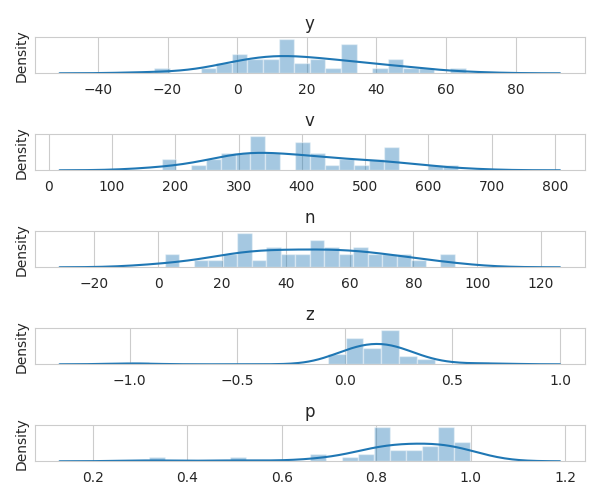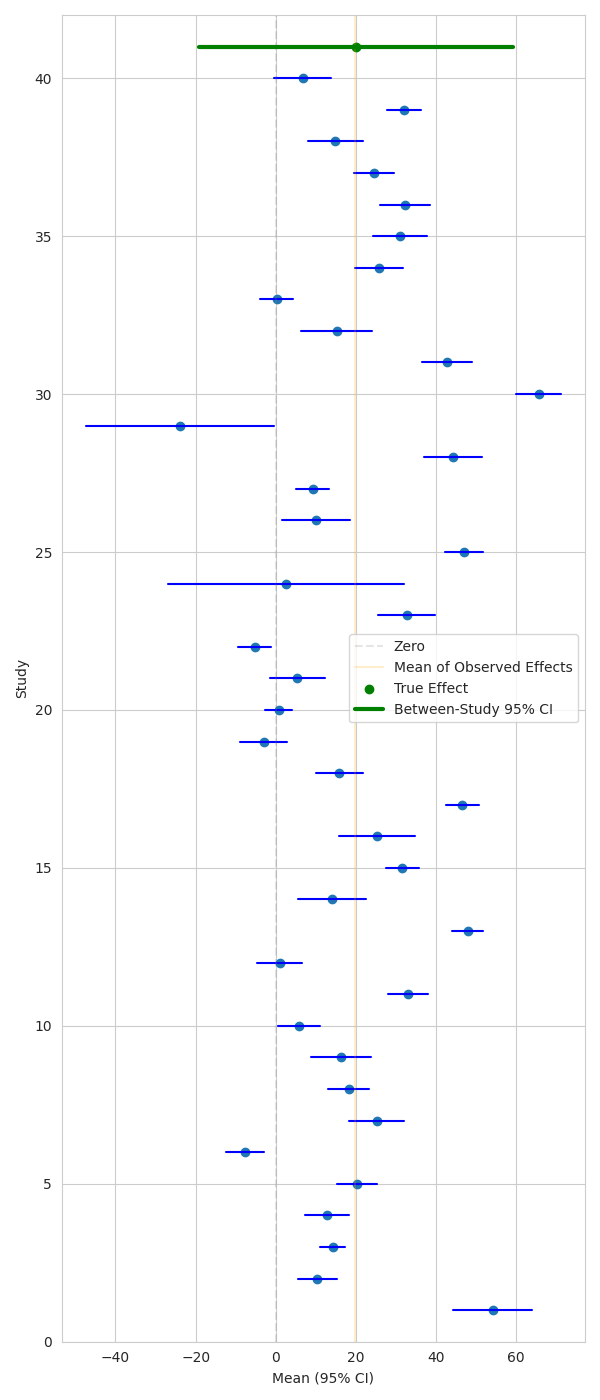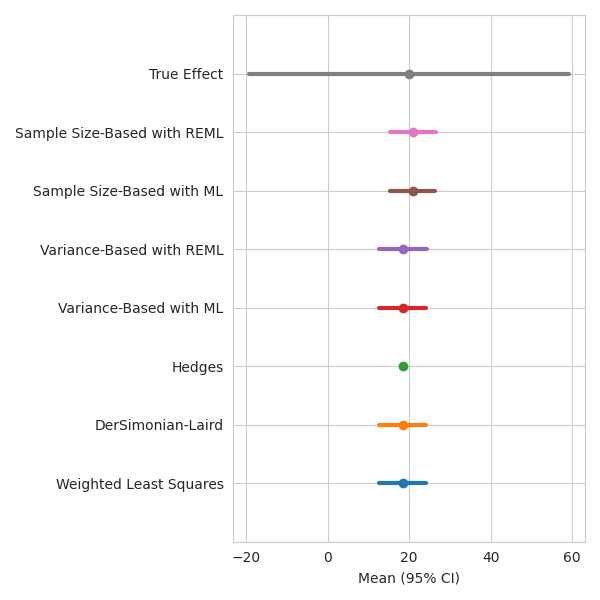# Run Estimators on a simulated dataset

PyMARE implements a range of meta-analytic estimators. In this example, we build a simulated dataset with a known ground truth and use it to compare PyMARE’s estimators.

Note

The variance of the true effect is composed of both between-study variance () and within-study variance (). Within-study variance is generally taken from sampling variance values from individual studies (v), while between-study variance can be estimated via a number of methods.

# sphinx_gallery_thumbnail_number = 3
import matplotlib.pyplot as plt
import numpy as np
import seaborn as sns
from scipy import stats

from pymare import core, estimators
from pymare.stats import var_to_ci

sns.set_style("whitegrid")


## Here we simulate a dataset

This is a simple dataset with a one-sample design. We are interested in estimating the true effect size from a set of one-sample studies.

N_STUDIES = 40
BETWEEN_STUDY_VAR = 400  # population variance
between_study_sd = np.sqrt(BETWEEN_STUDY_VAR)
TRUE_EFFECT = 20
sample_sizes = np.round(np.random.normal(loc=50, scale=20, size=N_STUDIES)).astype(int)
within_study_vars = np.random.normal(loc=400, scale=100, size=N_STUDIES)
study_means = np.random.normal(loc=TRUE_EFFECT, scale=between_study_sd, size=N_STUDIES)

sample_sizes[sample_sizes <= 1] = 2
within_study_vars = np.abs(within_study_vars)

# Convert data types and match PyMARE nomenclature
y = study_means
X = np.ones((N_STUDIES))
v = within_study_vars
n = sample_sizes
sd = np.sqrt(v * n)
z = y / sd
p = stats.norm.sf(abs(z)) * 2


## Plot variable distributions

fig, axes = plt.subplots(nrows=5, figsize=(6, 5))
sns.distplot(y, ax=axes, bins=20)
axes.set_title("y")
sns.distplot(v, ax=axes, bins=20)
axes.set_title("v")
sns.distplot(n, ax=axes, bins=20)
axes.set_title("n")
sns.distplot(z, ax=axes, bins=20)
axes.set_title("z")
sns.distplot(p, ax=axes, bins=20)
axes.set_title("p")
for i in range(5):
axes[i].set_yticks([])
fig.tight_layout()Out:

/home/docs/checkouts/readthedocs.org/user_builds/pymare/checkouts/latest/examples/02_meta-analysis/plot_meta-analysis_walkthrough.py:62: UserWarning:

distplot is a deprecated function and will be removed in seaborn v0.14.0.

Please adapt your code to use either displot (a figure-level function with
similar flexibility) or histplot (an axes-level function for histograms).

For a guide to updating your code to use the new functions, please see

sns.distplot(y, ax=axes, bins=20)

distplot is a deprecated function and will be removed in seaborn v0.14.0.

Please adapt your code to use either displot (a figure-level function with
similar flexibility) or histplot (an axes-level function for histograms).

For a guide to updating your code to use the new functions, please see

sns.distplot(v, ax=axes, bins=20)

distplot is a deprecated function and will be removed in seaborn v0.14.0.

Please adapt your code to use either displot (a figure-level function with
similar flexibility) or histplot (an axes-level function for histograms).

For a guide to updating your code to use the new functions, please see

sns.distplot(n, ax=axes, bins=20)

distplot is a deprecated function and will be removed in seaborn v0.14.0.

Please adapt your code to use either displot (a figure-level function with
similar flexibility) or histplot (an axes-level function for histograms).

For a guide to updating your code to use the new functions, please see

sns.distplot(z, ax=axes, bins=20)

distplot is a deprecated function and will be removed in seaborn v0.14.0.

Please adapt your code to use either displot (a figure-level function with
similar flexibility) or histplot (an axes-level function for histograms).

For a guide to updating your code to use the new functions, please see

sns.distplot(p, ax=axes, bins=20)


## Plot means and confidence intervals

Here we can show study-wise mean effect and CIs, along with the true effect and CI corresponding to the between-study variance.

fig, ax = plt.subplots(figsize=(6, 14))
study_ticks = np.arange(N_STUDIES)

# Get 95% CI for individual studies
lower_bounds, upper_bounds = var_to_ci(y, v, n)
ax.scatter(y, study_ticks + 1)
for study in study_ticks:
ax.plot((lower_bounds[study], upper_bounds[study]), (study + 1, study + 1), color="blue")
ax.axvline(0, color="gray", alpha=0.2, linestyle="--", label="Zero")
ax.axvline(np.mean(y), color="orange", alpha=0.2, label="Mean of Observed Effects")

# Get 95% CI for true effect
lower_bound, upper_bound = var_to_ci(TRUE_EFFECT, BETWEEN_STUDY_VAR, 1)
ax.scatter((TRUE_EFFECT,), (N_STUDIES + 1,), color="green", label="True Effect")
ax.plot(
(lower_bound, upper_bound),
(N_STUDIES + 1, N_STUDIES + 1),
color="green",
linewidth=3,
label="Between-Study 95% CI",
)
ax.set_ylim((0, N_STUDIES + 2))
ax.set_xlabel("Mean (95% CI)")
ax.set_ylabel("Study")
ax.legend()
fig.tight_layout()## Create a Dataset object containing the data

dset = core.Dataset(y=y, X=None, v=v, n=n, add_intercept=True)

# Here is a dictionary to house results across models
results = {}


## Fit models

When you have z or p:

When you have y and v and don’t want to estimate between-study variance:

When you have y and v and want to estimate between-study variance:

When you have y and n and want to estimate between-study variance:

When you have y and v and want a hierarchical model:

### First, we have “combination models”, which combine p and/or z values

The two combination models in PyMARE are Stouffer’s and Fisher’s Tests.

Notice that these models don’t use Dataset objects.

stouff = estimators.StoufferCombinationTest()
stouff.fit(z[:, None])
print("Stouffers")
print("p: {}".format(stouff.params_["p"]))
print()

fisher = estimators.FisherCombinationTest()
fisher.fit(z[:, None])
print("Fishers")
print("p: {}".format(fisher.params_["p"]))


Out:

Stouffers
p: [0.15549634]

Fishers
p: [0.85160016]


### Now we have a fixed effects model

This estimator does not attempt to estimate between-study variance. Instead, it takes tau2 () as an argument.

wls = estimators.WeightedLeastSquares()
wls.fit_dataset(dset)
wls_summary = wls.summary()
results["Weighted Least Squares"] = wls_summary.to_df()
print("Weighted Least Squares")
print(wls_summary.to_df().T)


Out:

Weighted Least Squares
0
name      intercept
estimate  18.610307
se         3.134726
z-score    5.936821
p-value         0.0
ci_0.025  12.466357
ci_0.975  24.754257


### Methods that estimate between-study variance

The DerSimonianLaird, Hedges, and VarianceBasedLikelihoodEstimator estimators all estimate between-study variance from the data, and use y and v.

DerSimonianLaird and Hedges use relatively simple methods for estimating between-study variance, while VarianceBasedLikelihoodEstimator can use either maximum-likelihood (ML) or restricted maximum-likelihood (REML) to iteratively estimate it.

dsl = estimators.DerSimonianLaird()
dsl.fit_dataset(dset)
dsl_summary = dsl.summary()
results["DerSimonian-Laird"] = dsl_summary.to_df()
print("DerSimonian-Laird")
print(dsl_summary.to_df().T)
print()

hedge = estimators.Hedges()
hedge.fit_dataset(dset)
hedge_summary = hedge.summary()
results["Hedges"] = hedge_summary.to_df()
print("Hedges")
print(hedge_summary.to_df().T)
print()

vb_ml = estimators.VarianceBasedLikelihoodEstimator(method="ML")
vb_ml.fit_dataset(dset)
vb_ml_summary = vb_ml.summary()
results["Variance-Based with ML"] = vb_ml_summary.to_df()
print("Variance-Based with ML")
print(vb_ml_summary.to_df().T)
print()

vb_reml = estimators.VarianceBasedLikelihoodEstimator(method="REML")
vb_reml.fit_dataset(dset)
vb_reml_summary = vb_reml.summary()
results["Variance-Based with REML"] = vb_reml_summary.to_df()
print("Variance-Based with REML")
print(vb_reml_summary.to_df().T)
print()

# The SampleSizeBasedLikelihoodEstimator estimates between-study variance
# using y and n, but assumes within-study variance is homogenous
# across studies.
sb_ml = estimators.SampleSizeBasedLikelihoodEstimator(method="ML")
sb_ml.fit_dataset(dset)
sb_ml_summary = sb_ml.summary()
results["Sample Size-Based with ML"] = sb_ml_summary.to_df()
print("Sample Size-Based with ML")
print(sb_ml_summary.to_df().T)
print()

sb_reml = estimators.SampleSizeBasedLikelihoodEstimator(method="REML")
sb_reml.fit_dataset(dset)
sb_reml_summary = sb_reml.summary()
results["Sample Size-Based with REML"] = sb_reml_summary.to_df()
print("Sample Size-Based with REML")
print(sb_reml_summary.to_df().T)


Out:

DerSimonian-Laird
0
name      intercept
estimate   18.65147
se         3.177585
z-score    5.869699
p-value         0.0
ci_0.025  12.423517
ci_0.975  24.879423

Hedges
0
name       intercept
estimate   18.610307
se          0.158114
z-score   117.701915
p-value          0.0
ci_0.025   18.300409
ci_0.975   18.920204

Variance-Based with ML
0
name      intercept
estimate  18.717711
se         3.250726
z-score     5.75801
p-value         0.0
ci_0.025  12.346405
ci_0.975  25.089016

Variance-Based with REML
0
name      intercept
estimate  18.751613
se         3.290303
z-score    5.699053
p-value         0.0
ci_0.025  12.302737
ci_0.975   25.20049

Sample Size-Based with ML
0
name      intercept
estimate  20.049697
se         3.063823
z-score    6.544013
p-value         0.0
ci_0.025  14.044714
ci_0.975  26.054679

Sample Size-Based with REML
0
name      intercept
estimate  20.049667
se          3.10282
z-score    6.461756
p-value         0.0
ci_0.025  13.968251
ci_0.975  26.131082


### What about the Stan estimator?

We’re going to skip this one here because of how computationally intensive it is.

### Let’s check out our results!

fig, ax = plt.subplots(figsize=(6, 6))

for i, (estimator_name, summary_df) in enumerate(results.items()):
ax.scatter((summary_df.loc[0, "estimate"],), (i + 1,), label=estimator_name)
ax.plot(
(summary_df.loc[0, "ci_0.025"], summary_df.loc[0, "ci_0.975"]),
(i + 1, i + 1),
linewidth=3,
)

# Get 95% CI for true effect
lower_bound, upper_bound = var_to_ci(TRUE_EFFECT, BETWEEN_STUDY_VAR, 1)
ax.scatter((TRUE_EFFECT,), (i + 2,), label="True Effect")
ax.plot(
(lower_bound, upper_bound),
(i + 2, i + 2),
linewidth=3,
label="Between-Study 95% CI",
)
ax.set_ylim((0, i + 3))
ax.set_yticklabels([None] + list(results.keys()) + ["True Effect"])

ax.set_xlabel("Mean (95% CI)")
fig.tight_layout()Out:

/home/docs/checkouts/readthedocs.org/user_builds/pymare/checkouts/latest/examples/02_meta-analysis/plot_meta-analysis_walkthrough.py:261: UserWarning: FixedFormatter should only be used together with FixedLocator
ax.set_yticklabels([None] + list(results.keys()) + ["True Effect"])


Total running time of the script: ( 0 minutes 1.371 seconds)

Gallery generated by Sphinx-Gallery